# C++ Program for Binary Search

In this article, you will learn and get code for searching for an element in an array using the binary search technique in C++ programming. Here are the approaches used:

Before going through the above programs, if you're not aware of the binary search technique, you can refer to binary search to understand its logic and algorithm.

## Binary Search in C++

To search an element from an array using the binary search technique in C++ programming, you have to ask the user to enter any 10 elements for the array and then enter the element or number to be searched.

After searching the element using the binary search technique, if it is available in the list, display the position of the element. The following C++ program asks the user to enter any 10 array elements and an element to be searched. Here is the simple binary search program:

```#include<iostream>
using namespace std;
int main()
{
int i, arr, num, first, last, middle;
cout<<"Enter 10 Elements (in ascending order): ";
for(i=0; i<10; i++)
cin>>arr[i];
cout<<"\nEnter Element to be Search: ";
cin>>num;
first = 0;
last = 9;
middle = (first+last)/2;
while(first <= last)
{
if(arr[middle]<num)
first = middle+1;
else if(arr[middle]==num)
{
cout<<"\nThe number, "<<num<<" found at Position "<<middle+1;
break;
}
else
last = middle-1;
middle = (first+last)/2;
}
if(first>last)
cout<<endl;
return 0;
}```

This program was built and runs under the Code::Blocks IDE. Here is the initial snapshot of the sample run: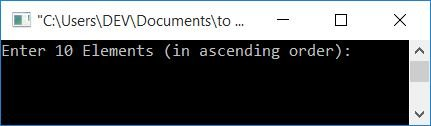Now enter any 10 numbers (in ascending order) as array elements, say 1, 2, 3, 4, 5, 6, 7, 8, 9, and 10, and then a number that is going to be searched from the list, say 8. Here is the final snapshot of the sample run: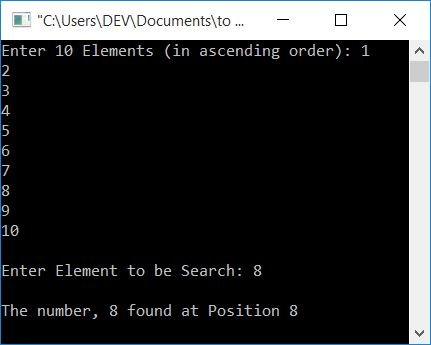If the user enters 10 elements as 1, 2, 3, 4, 5, 6, 7, 8, 9, 10, and the element to be searched as 8, the above program will run as follows:

• 10 elements get stored in the array arr[] in a way that arr = 1, arr = 2, arr = 3, and so on.
• The number to be searched, say 8, gets initialized to num. Therefore, num=8
• Initially, first = 0 and last = 9.
• (first+last)/2 or 0+9/2 or 4 gets initialized to middle. Therefore middle=4
• Because the value of first (0) is less than the value of last (9), the condition of the while loop evaluates to be true. Therefore, program flow goes inside the loop
• checks whether arr[middle]<num evaluates to be true or not. When the values of variables middle and num are entered, arr<num or 5<8 evaluates to be true. As a result, program flow enters the if block, and middle+1, 4+1, or 5 is set to first.
• Because the condition of the if block evaluates to true, the statement(s) of the else if and else block will not be executed.
• At last, middle = (first+last)/2, or middle = (5+9)/2, or middle=7.
• Program flow goes back to the condition of the while loop. The condition first <= last, or 5<=9 again evaluates to be true; therefore, again, program flow goes inside the loop.
• Checks whether the if block's condition, arr[middle]<num, evaluates to true or false, that is, arr<num or 8<8 evaluates to false. As a result, program flow proceeds to the else if block and checks its condition.
• The condition arr[middle]==num or 8==8 is satisfied. Therefore, program flow goes inside this else if block and executes its statements.
• That is, it prints the position of the number, because the number is found at index number 7. Therefore, I've printed its position as 8, since indexing in arrays starts from 0, not from 1.
• After printing the position of the element, use the break keyword to break out of the while loop.
• After exiting the while loop, check the condition using the if block, that is, whether first's value is greater than the value of last or not.
• If condition is true, print a message indicating that the number was not found in the list.

### Allow the user to define the size

This program allows the user to define the size of the array. It also doesn't care about the way the user enters the data, that is, either in ascending or random order. Because, after receiving all the numbers, we've created a block of code that sorts the list in ascending order (before performing the search operation). Then, as shown in the program below, ask for an element to be searched from the sorted list:

```#include<iostream>
using namespace std;
int main()
{
int len, i, arr, num, j, temp, first, last, middle;
cout<<"Enter the Size: ";
cin>>len;
cout<<"Enter "<<len<<" Elements: ";
for(i=0; i<len; i++)
cin>>arr[i];
// sort the array first
for(i=0; i<(len-1); i++)
{
for(j=0; j<(len-i-1); j++)
{
if(arr[j]>arr[j+1])
{
temp = arr[j];
arr[j] = arr[j+1];
arr[j+1] = temp;
}
}
}
// print the sorted array
cout<<"\nThe New Sorted Array is:\n";
for(i=0; i<len; i++)
cout<<arr[i]<<" ";
// now back to binary search
cout<<"\n\nEnter Element to be Search: ";
cin>>num;
first = 0;
last = (len-1);
middle = (first+last)/2;
while(first <= last)
{
if(arr[middle]<num)
first = middle+1;
else if(arr[middle]==num)
{
cout<<"\nThe number, "<<num<<" found at Position "<<middle+1;
break;
}
else
last = middle-1;
middle = (first+last)/2;
}
if(first>last)
cout<<endl;
return 0;
}```

Here is its sample run, supposing that the user enters the array size as 5 and its elements as 5, 1, 4, 2, and 3: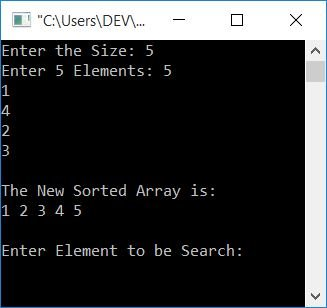Now enter an element, say 4, to search it from the list, and print its position as shown in the output given below: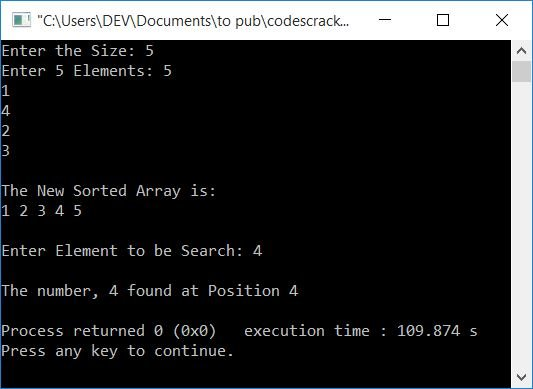## Binary search in C++, using user-defined functions

This program is created using a user-defined function, binSearRecFun(), that receives three arguments. The first is the array, the second is the number to be searched, and the third is the array's size.This function either returns the position of the element in the list or a value of 0 that indicates the number is not available in the list.

Before the binary search, there is also a function called sortArray() that sorts the given array in ascending order.

```#include<iostream>
using namespace std;
void sortArray(int [], int);
int binSearchFun(int [], int, int);
int main()
{
int len, i, arr, num, pos;
cout<<"Enter the Size (max. 50): ";
cin>>len;
cout<<"Enter "<<len<<" Elements: ";
for(i=0; i<len; i++)
cin>>arr[i];
// sort the array first
sortArray(arr, len);
// print the sorted array
cout<<"\nThe New Sorted Array is:\n";
for(i=0; i<len; i++)
cout<<arr[i]<<" ";
cout<<"\n\nEnter Element to be Search: ";
cin>>num;
// search element using binary search
pos = binSearchFun(arr, num, len);
if(pos==0)
cout<<endl<<num<<" isn't available in the list";
else
cout<<endl<<num<<" is available at Position "<<pos;
cout<<endl;
return 0;
}
void sortArray(int arr[], int len)
{
int i, j, temp;
for(i=0; i<(len-1); i++)
{
for(j=0; j<(len-i-1); j++)
{
if(arr[j]>arr[j+1])
{
temp = arr[j];
arr[j] = arr[j+1];
arr[j+1] = temp;
}
}
}
}
int binSearchFun(int arr[], int num, int len)
{
int first, last, middle;
first = 0;
last = (len-1);
middle = (first+last)/2;
while(first <= last)
{
if(arr[middle]<num)
first = middle+1;
else if(arr[middle]==num)
return (middle+1);
else
last = middle-1;
middle = (first+last)/2;
}
return 0;
}```

Here is its sample run, supposing that the user enters the size as 6, its elements as 6, 1, 5, 2, 3, and 4, and the number to be searched as 4: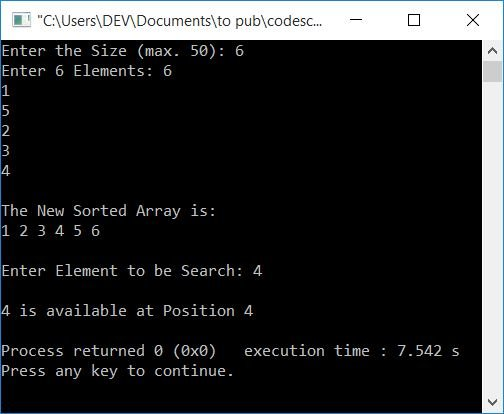## Binary search in C++ using recursion

This program uses recursion (a function that calls itself) to search an element using the binary search technique.

```#include<iostream>
using namespace std;
int binSearRecFun(int [], int, int, int);
int main()
{
int i, arr, num, pos;
cout<<"Enter 10 elements (in ascending order): ";
for(i=0; i<10; i++)
cin>>arr[i];
cout<<"\nEnter element to be search: ";
cin>>num;
pos = binSearRecFun(arr, 0, 9, num);
if(pos==0)
cout<<endl<<num<<" is not available in the list";
else
cout<<endl<<num<<" is available at Position "<<pos;
cout<<endl;
return 0;
}
int binSearRecFun(int arr[], int first, int last, int num)
{
int middle;
if(first>last)
return 0;
middle = (first+last)/2;
if(arr[middle]==num)
return (middle+1);
else if(arr[middle]>num)
binSearRecFun(arr, first, middle-1, num);
else if(arr[middle]<num)
binSearRecFun(arr, middle+1, last, num);
}```

Here is its sample run: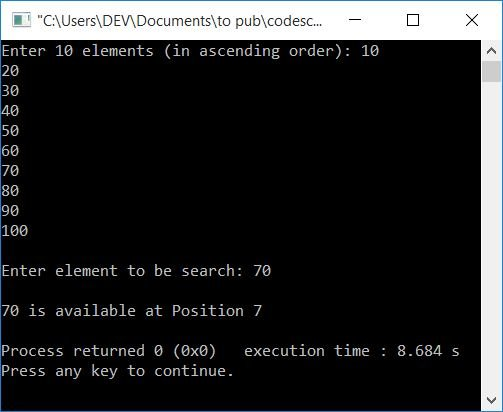#### The same program in different languages

C++ Quiz

« Previous Program Next Program »